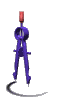Einstein's Mass-Energy Equivalence Original Post: 22 June 2012 Posted Here: 4 December 2017For years we have heard that Einstein's famous equation, e = mc^2, says that in a nuclear reaction, such as the fission of uranium-235 in a nuclear power plant, the fusion of hydrogen that powers a star, or radioactive decay, mass is converted into energy. This is sometimes even demonstrated by showing that the masses of the products are less than of the reactants. Since the assertion was always made by some authority figure, we assumed it was true. Not a good modus operandi for any reader of science. Einstein's equation does not say that mass can be converted into energy (or that energy can be converted into mass.) I thank Richard S. Treplow, in “E=mc^2 for the Chemist: When is Mass Conserved?”, J. Chem. Ed., Nov. 2005, pg. 1636, for first pointing this out to me. Contrary to popular belief, Einstein's equation does not imply that mass and energy are interconvertible. A (fictitious) equation which permits such conversions would be of a form like m + e = c^2. The equation that describes the relationship between kinetic and potential energies is an example of that type of equation: ek + ep = et. Kinetic energy (ek) plus potential energy (ep) equals the total energy (et). Kinetic energy can be converted to potential energy (ek becomes smaller while ep becomes larger) or vise versa because their sum is a constant (et.) Einstein's equation says the opposite is true of mass and energy: It says that if energy increases then mass must also increase and if energy decreases then mass must also decrease, because, according to the equation, mass and energy are directly proportional.A familiar relationship described by an equation of the form e = mc^2 is the equation for the circumference of a circle: C = D(pi). Circumference equals pi times the diameter. If mass can be converted into energy (and vise versa), then diameter can be converted into circumference (and vise versa). When was the last time you heard someone say that decreasing the diameter would increase the circumference of a circle or that decreasing the circumference would increase the diameter? Let's measure off a piece of rope 31.4 feet long and lay it onto the ground to form a circle. If we measure it (or use the equation C = D(pi),) the diameter of this circle is 10 feet. Let's call this our "reactant" circle, because we want to remove ("release") some circumference to form a "product" circle: Cut out a piece of rope 3.14 feet long and reform the circle with the remaining 28.26 feet of rope. This product circle now has a diameter of 9 feet. According to some physicists, we have converted one foot of diameter from the reactant circle into 3.14 feet of circumference (represented by the piece of rope dangling from our hand.) But, wait a minute! We have this chunk of rope 3.14 feet long, but it doesn't represent any circumference. Okay, let's join the ends to make a little circle of circumference 3.14 feet and, voila!, it has a diameter of 1 foot. That one foot of diameter that's missing from our product circle wasn't used to make the circumference of this circle, it's still here as the diameter of the little circle. Similarly, when energy is removed ("released") from the nucleus of an atom, the mass which "disappears" was not used to create this energy. It goes right along with the released energy, just as diameter goes right along with circumference. So why does the mass seem to disappear? The energy certainly doesn't. The answer lies in the difference between the equations' constants: pi vs. c squared. Because the constant, pi, equals 3.14, diameter is about a third of circumference--they have similar sizes. So a circle of noticeable circumference will have a measurable diameter. But the constant in Einstein's equation--c squared--is an extremely large number. Unless the amount of energy we are observing is also tremendously large, like the output of a star, the mass which accompanies the puny amount of energy released by any nuclear reaction seen on Earth will be, for practical purposes, immeasurable. (Yes, I'm saying that the energy released by a nuclear reactor or a hydrogen bomb is "puny"--when compared to the energy output of any star.) Okay, for practical purposes, the mass released is immeasurable. But we could still ask, "Where is the mass?" "Everyone knows" that the reason a spaceship cannot exceed the speed of light is because its mass increases toward infinity as its velocity increases toward light speed. Its velocity increases because we have transferred energy from some chemical or physical source to the ship's kinetic energy--it moves faster. When the energy of a nuclear reaction is transferred to other atoms and molecules in the environment, their velocity likewise increases (but to nowhere near the speed of light.) This means that their mass increases, just like the starship's. The energy and velocities of the environmental atoms and molecules is readily noticeable but, because of the magnitude of c squared, their increase in mass is not, just as we don't notice the increase in mass of a spaceship like the shuttle as it travels at orbital velocities. For nuclear reactions, mass seems to have disappeared, but it hasn't. It's just unnoticed. Mass and energy: They are like the horse and carriage--You can't have one without the other. And you can't convert one into the other, either. One last little matter: Circumference and diameter are two things related by a direct proportion that describes a circle. Mass and energy are two things related by a direct proportion that describe a…??? Well, we know what a circle is, but do we know what it is that mass and energy describe? [Could they describe information? (This suggestions is added on 11 December 2015.)]